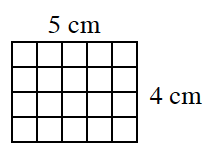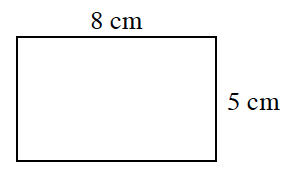### Home > CC1MN > Chapter 1 Unit 1 > Lesson CC1: 1.1.2 > Problem1-11

1-11.

In the “Toothpick and Tiles” game, you looked at the number of square tiles and the number of toothpicks used to form shapes. The math words that describe the number of tiles and toothpicks are area and perimeter. Read the Math Notes box for this lesson to review how area and perimeter are related to tiles and toothpicks. Then follow the directions below.

1. Find the area and perimeter of the tile figure at right.Count the squares in the rectangle to find the area. How does the number of squares relate to the base and height?

Try adding up all the sides of the rectangle to find the perimeter: 5 cm + 5 cm + 4 cm + 4 cm

1. Find the area and perimeter of the rectangle at right.See part (a). The area is the number of square units in a shape. The perimeter is the total length of all the sides.

Area: 40 sq cm
Perimeter: 26 cm

Be sure to explain how these answers relate to the given measurements of the rectangle.

1. Now design your own shape with 5 square tiles. Record the perimeter and the area.

Use the eTool below to explore area and perimeter that can be created with 5 square tiles.
Click on the link at right for the full eTool version: CC1 1-11 HW eTool